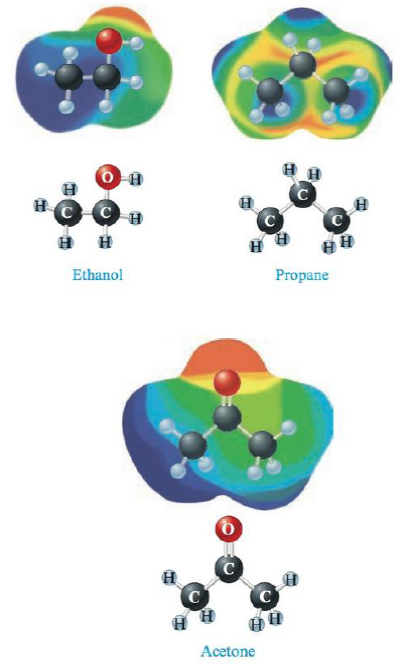# Consider the following electrostatic potential diagrams: Rank the compounds from lowest to highest boiling point and explain your answer.### Chemistry: An Atoms First Approach

2nd Edition
Steven S. Zumdahl + 1 other
Publisher: Cengage Learning
ISBN: 9781305079243

#### Solutions

Chapter
Section### Chemistry: An Atoms First Approach

2nd Edition
Steven S. Zumdahl + 1 other
Publisher: Cengage Learning
ISBN: 9781305079243
Chapter 9, Problem 40E
Textbook Problem
12 views

## Consider the following electrostatic potential diagrams:Rank the compounds from lowest to highest boiling point and explain your answer.

Interpretation Introduction

Interpretation:

The boiling point of the given compounds are should be explained.

Concept Introduction:

Intermolecular forces:

• The forces involving within the molecule is called intermolecular forces thus,

Ion-dipole forces:

The attraction forces between anion charge end with opposite partial charge is called Ion-dipole forces.

Dipole- Dipole forces:

The attraction forces between ends of opposite partially charge in polar molecules is called Dipole- Dipole forces.

Hydrogen bond:

The weak bond between highly electronegative atom with partially positively charged Hydrogen is called Hydrogen bonding.

London Dispersion Forces:

The London dispersion force is the weaker force within the non polar molecules and which can be used to explain the state of the non polar molecules.

• The intermolecular forces are high means the boiling point of the compound is all so high.

### Explanation of Solution

Explanation

The given electrostatic potential diagrams are recorded as shown above.

To explain the boiling points of given compounds.

• Ethanol and acetone are polar molecules and propane is a non polar molecule in given electrostatic potential diagrams (Fig 1).

For Ethanol,

• Ethanol exhibit strong hydrogen bonding and London dispersion forces so it will have more boiling point than Acetone and Propane...

### Still sussing out bartleby?

Check out a sample textbook solution.

See a sample solution

#### The Solution to Your Study Problems

Bartleby provides explanations to thousands of textbook problems written by our experts, many with advanced degrees!

Get Started